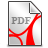# Plemelj's extended Green identities

Let du/dn denote the directional derivative of u in the direction of the outward pointing normal n to the surface element. An uniquely defined and continuous normal n enables a meaningful definition of du/dn, which is one central object in the Green identities.

The proofs of the Green identities are based on the assumption, that the potential together with its first derivatives is finite and continuous to the boundary, whereby a smooth continuation to the continuous boundary values is given.

The alternatively proposed definition from Plemelj of the directional derivative of u on the boundary enables an extension of the Green identities with reduced regularity assumptions to the potential function on the boundary, where the normal derivative is replaced by a differential of the conjugate potential function (in case of space dimension n=2), with the following reduced regularity assumptions:

the normal derivative of a potential function u needs no longer to be existing everywhere on the boundary, but only the conjugate function of this potential u is required to be continuous on the boundary. This compares to an extention from differentiable functions to continuous functions. The extended Green formulas are (in a nutshell) given as follows ((PlJ) p. 9-13):

let D(u,v) denote the Dirichlet integral (inner product) with domain D and let <u,v> denote the related inner product on the boundary of D. Let further u denote the conjugate function of a potential function u. Then it holds:

i)  D(u,u)=<u,du> is positive for all non constant potential functions u
ii) <v,du>=<u,dv>

By operating with "div" on both sides of the NSE the field p(x,t) can be formally obtained as a solution of a Neumann problem. Therefore, to describe the values of the pressure at the bounding walls or at the initial time independently of v, could incompatible with the NSE and, therefore, could render the problem ill-posed. The above extended Green formulas provide a framework to overcome this Neumann "pressure" incompatibility problem.

Let ((v,w)) denote the inner product of the Hilbert space H(-1/2). Then the Plemelj extension of the Green formula enables a (weak) variation representation of the potential equation with respect this inner product, whereby the corresponding energy norm is given by the norm of the Hilbert space H(1/2).

In other words: Plemelj’s extension of the Green identities enables an extended domain H(1/2) of the corresponding “energy”inner product, which is derived from the Dirichlet integral.

In the fluid theory beside the Laplacian and the Gradient operator the following operators play a key role:

1. the Trace operator div(u)

2. the Vorticity operator curl(u)

3. the Helicity operator u*curl(u).

The identity

enables a properly defined (Plemelj) directional derivative of  u(,grad)u on the boundary.

For the following we refer to the below paper from Y. Giga (GiY). Let n=3 and p=2, then it holds:

1. (GiY), Sobolev embedding theorem:

the Hilbert space H(1/2) is a subset of L(3)

2. (GiY) Lemma 3.2:

Let r=3, “delta”=3/4, “theta”=”roh”=1/4 then the absolute value of the trilinear form    ((P(u,grad)v,w))    is uniformly bounded with respect to the H(1/2)-norms of  u,v and w.

We claim that as a consequence of the above a weak representation of the NSE with respect to the inner product of H(-1/2) is well posed, i.e. there exists an unique solution, which is continuously depending from the initial and boundary values.

The semigroup estimates ((GiY), Lemma 2.1, see also Nitsche J. A., approximation theory in Hilbert scale; extensions) indicate the to be defined appropriate exponent of the time variable in order to achieve  a balance between the above H(-1/2) variation representation of the NSE and a corresponding time-weighted H(0) variation representation with "standard" regularity requirements to the potential.Giga Y., Weak and strong solutions of the Navier-Stokes initial value problem.pdf (2.06MB)Giga Y., Weak and strong solutions of the Navier-Stokes initial value problem.pdf (2.06MB)Fujita H., Morimoto H., On Fractional Powers of the Stokes Operator.pdf (291.3KB)Fujita H., Morimoto H., On Fractional Powers of the Stokes Operator.pdf (291.3KB)Nitsche J. A., lecture notes, Hilbert scale, extensions, approximation theory.pdf (382.6KB)Nitsche J. A., lecture notes, Hilbert scale, extensions, approximation theory.pdf (382.6KB)